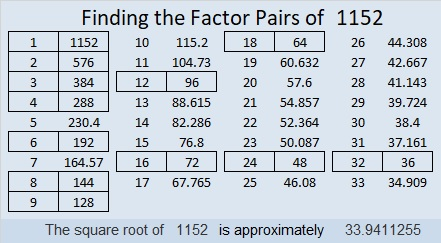# 1152 Will You See All the Prime Factors in This Factor Tree?

Will you see all the prime factors in this factor tree when all of the factors are the same color? You know how to count, but would you possibly not count one of the prime factors or possibly count one of them twice?Is it easier to count the prime factors in the following factor trees?If you happen to have two different colors of ink and/or pencils around when you make factor trees, they might be easier to read especially if the factored number has a lot of factors like 1152 does.

Here’s what I’ve learned about the number 1152:

• 1152 is a composite number.
• Prime factorization: 1152 = 2 × 2 × 2 × 2 × 2 × 2 × 2 × 3 × 3, which can be written 1152 = 2⁷ × 3²
• The exponents in the prime factorization are 7 and 2. Adding one to each and multiplying we get (7 + 1)(2 + 1) = 8 × 3 = 24. Therefore 1152 has exactly 24 factors.
• Factors of 1152: 1, 2, 3, 4, 6, 8, 9, 12, 16, 18, 24, 32, 36, 48, 64, 72, 96, 128, 144, 192, 288, 384, 576, 1152
• Factor pairs: 1152 = 1 × 1152, 2 × 576, 3 × 384, 4 × 288, 6 × 192, 8 × 144, 9 × 128, 12 × 96, 16 × 72, 18 × 64, 24 × 48, or 32 × 36
• Taking the factor pair with the largest square number factor, we get √1152 = (√576)(√2) = 24√2 ≈ 33.94112The last two digits of 1152 are 52, a number divisible by 4, so 1152 can be evenly divided by 4.
1 + 1 + 5 + 2 = 9, so 1152 is divisible by 9.

It is so easy to tell if a number can be evenly divided by 4 or 9 AND it is so easy to divide by 4 or by 9. When I make a factor cake, I like to see if the current layer of the cake is divisible by 4 or by 9 before I check to see if it is divisible by a prime number.From that cake, I can quickly tell that 1152 = 2⁷ × 3² by simply counting by 2’s to find the powers of 2 and 3. All the numbers being the same color doesn’t even slow me down.

I can also easily find the √1152 by taking the square root of everything on the outside of the cake:
√1152  = √(4·4) · (√4)(√9)(√2) = (4·2·3)√2 = 24√2

Since MOST square roots that can be simplified are divisible by 4, or by 9, or by both, this is a good strategy to find their square roots.

Here are some more facts about this number:

1152 is the sum of the fourteen prime numbers from 53 to 109,
and it is the sum of the twelve prime numbers from 71 to 127.

34² – 2² = 1152 so we are only 2² = 4 numbers away from the next perfect square.

1152 looks interesting when it is written in these bases:
It’s 800 in BASE 12 because 8(12²) = 1152,
242 in BASE 23 because 2(23²) + 4(23) + 2(1) = 1152
200 in BASE 24 because 2(24²) = 1152,
WW in BASE 35 (W is 32 base 10) because 32(35) + 32(1) = 32(36) = 1152, and
it’s W0 in BASE 36 because 32(36) = 1152

This site uses Akismet to reduce spam. Learn how your comment data is processed.